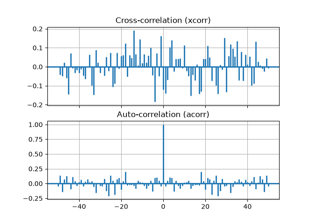# matplotlib.axes.Axes.xcorr#

Axes.xcorr(x, y, normed=True, detrend=<function detrend_none>, usevlines=True, maxlags=10, *, data=None, **kwargs)[source]#

Plot the cross correlation between x and y.

The correlation with lag k is defined as $$\sum_n x[n+k] \cdot y^*[n]$$, where $$y^*$$ is the complex conjugate of $$y$$.

Parameters:
x, yarray-like of length n
detrendcallable, default: mlab.detrend_none (no detrending)

A detrending function applied to x and y. It must have the signature

detrend(x: np.ndarray) -> np.ndarray

normedbool, default: True

If True, input vectors are normalised to unit length.

usevlinesbool, default: True

Determines the plot style.

If True, vertical lines are plotted from 0 to the xcorr value using Axes.vlines. Additionally, a horizontal line is plotted at y=0 using Axes.axhline.

If False, markers are plotted at the xcorr values using Axes.plot.

maxlagsint, default: 10

Number of lags to show. If None, will return all 2 * len(x) - 1 lags.

Returns:
lagsarray (length 2*maxlags+1)

The lag vector.

carray (length 2*maxlags+1)

The auto correlation vector.

line

Artist added to the Axes of the correlation:

bLine2D or None

Horizontal line at 0 if usevlines is True None usevlines is False.

Other Parameters:
linestyleLine2D property, optional

The linestyle for plotting the data points. Only used if usevlines is False.

markerstr, default: 'o'

The marker for plotting the data points. Only used if usevlines is False.

dataindexable object, optional

If given, the following parameters also accept a string s, which is interpreted as data[s] (unless this raises an exception):

x, y

**kwargs

Additional parameters are passed to Axes.vlines and Axes.axhline if usevlines is True; otherwise they are passed to Axes.plot.

Notes

The cross correlation is performed with numpy.correlate with mode = "full".

## Examples using matplotlib.axes.Axes.xcorr#Cross- and auto-correlation

Cross- and auto-correlation